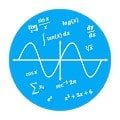# Calculator N+ (Open source) – Math Solver APK

The Calculator provides the following features:
ALGEBRA
1. Computer science
2. Solving the equation:
3. Solve system equations
4. Graphing
5. Cartesian geometry sand(Viet Nam Education)
6. Unit Conversions
8. Simplify expressions
9. Polynomial factorization.
10. Binomial expansion Newton
11. Matrix: evalute matrix step by step

ANALYTICS
1. Derivative
2. Antiderivative
3. Definite integrals
4. Find the limit of the sequence, function

TRIGONOMETRIC
1, Trigonometric expand: sin(2x) -> 2sin(x)cos(x)
2. Trigonometric reduce: 2sin(x)cos(x) -> sin(2x)
3. Trigonometric to exponent: sinh(x) -> (e^x-e^(-x))/2

DETERMINATION OF STATISTICS
1. Combination
2. Permutation

SOME OTHER FEATURES
1. Prime factors
2. Modulo
3. Catalan number
4. Fibonacci number

The Calculator does not show the steps to solve the equations, system of equations, derivative… but only for the final result.
Enter sin(30°) to calculate sine Degree mode

The computer can work in two modes:
– Decimal mode: 0.12312312323
– Mode fractions: for results with arbitrary precision, for example 9^99999

Any suggestions on the application please send mail: [email protected]

See in github Github.com/tranleduy2000/ncalc

#### What’s New

Update math engine and documents
IDE mode